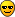# Fit with unsymmetrical gauss function

Hallo!
I have to fit some peaks with a gauss function on quadratic background, so I’ve defined:

Double_t gaus_lau (Double_t *x, Double_t *par)

{

``````  Double_t pi = 3.1415926535;

Double_t xx =x;

return par*exp(-(pow(((xx-par)/par),2))/2) + par + par*xx + par * xx * xx;
``````

}

The problem now is that these peaks have a tail on one side, so I would like to use an unsymmetrical function…something like a gauss function with 2 different FWHM…but I don’t know how to do thiscan you help me? Thank you!

Hi Laly86,

Try something along the following lines:

``````Double_t gaus_lau (Double_t *x, Double_t *par)
{
static Double_t pi = 3.1415926535;

const Double_t xx =x;

const Double_t width = (xx > par) ? par : par;
const Double_t arg    = pow(((xx-par)/width),2);
const Double_t ampl  = par;

return ampl*exp(-arg/2) + par + xx*(par + xx * par);
}``````

Hi,

Actually FWHM is proportional to sigma ( par in your function ).
It is needed to add another parameter, since you have two sigmas.
In the following there is an example how to that,.
Maybe there are simpler ways also, but I don’t know…

``````#include <TF1.h>

double Gaus_2sigma( double *, double* );

void Gaus_2sigmas()
{

TF1 *f_Gaus_2sigma = new TF1("f_Gaus_2sigma", Gaus_2sigma, -5, 5, 7 );
f_Gaus_2sigma->SetParameters(100, 0, 0.5, 1.5, 0, 0, 0);
f_Gaus_2sigma->Draw();

}

double Gaus_2sigma( double *x, double*par )
{
double xx = x;
double height = par;
double mean = par;
double sigma_1 = par;
double sigma_2 = par;

double Pol = par + xx*(par + xx*par );

if( xx < mean )
{
return Pol + height*exp( -(xx - mean)*(xx - mean)/(2*sigma_1*sigma_1) );
}
else
{
return Pol + height*exp( -(xx - mean)*(xx - mean)/(2*sigma_2*sigma_2) );
}
}``````

I’ve tried your functions, they’re both perfect for my fit!Thank you very much!